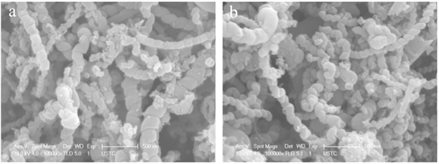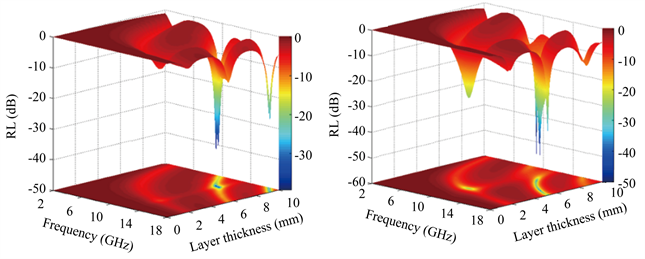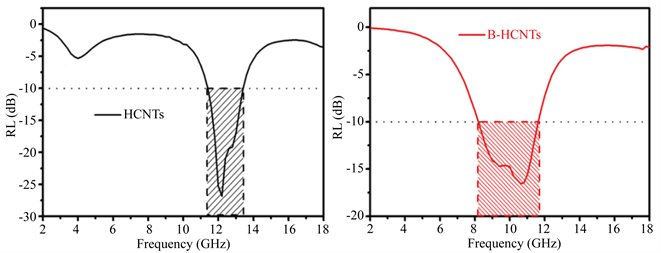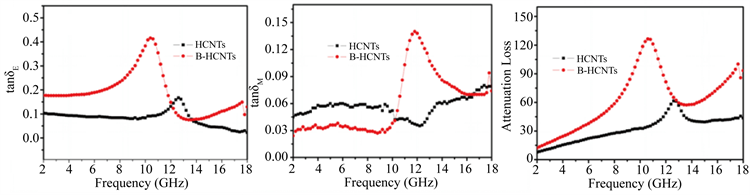#### 期刊菜单

Effect of Boron Doping on Microwave Absorption Properties of Helical Carbon Nanotubes
DOI: 10.12677/MP.2021.113007, PDF, HTML, XML, 下载: 185  浏览: 477  科研立项经费支持

Abstract: Boron-doped helical carbon nanotubes (B-HCNTs) were synthesized by thermal annealing helical carbonnanotubes (HCNTs) in the presence of boric oxid. The investigation of FE-SEM revealed that the structures of HCNTs were well retained during the boron doping process, and XPS analysis demonstrated that the boron atoms were indeed doped in the structure of HCNTs. The as-prepared B-HCNTs displayed superior electromagnetic wave absorption capabilities in terms of the optimal reflection loss value of −50.19 dB and the absorption bandwidth of 3.4 GHz. And the enhanced EMW absorption capabilities of B-HCNTs were analyzed in details. Considering the simple process, low density and high chemical stability, the designed B-HCNTs samples are very promising candidates for lightweight and high-efficiency microwave absorption materials.

1. 引言

2. 实验

3. 表征与讨论Figure 1. FE-SEM images of HCNTs (a) and B-HCNTs (b)Figure 2. XPS spectra of HCNTs (a) and B-HCNTs (b)

${\epsilon }^{\prime }={\epsilon }_{\infty }+\frac{{\epsilon }_{s}-{\epsilon }_{\infty }}{1+{\omega }^{2}{\tau }^{2}}$ (1)

${\epsilon }^{″}=\frac{{\epsilon }_{s}-{\epsilon }_{\infty }}{1+{\omega }^{2}{\tau }^{2}}\omega \tau +\frac{{\sigma }_{ac}}{\omega {\epsilon }_{0}}$ (2)Figure 3. Complex permittivity (a), (b) and permeability (c), (d) for the pure and B-doped HCNTs as a function of frequency

${Z}_{in}={Z}_{0}\sqrt{\frac{\mu }{\epsilon }}\mathrm{tanh}\left(j\frac{2\pi fd\sqrt{\mu \epsilon }}{c}\right)$ (3)

$\text{RL}=20\mathrm{log}|\frac{{Z}_{in}-{Z}_{0}}{{Z}_{in}+{Z}_{0}}|$ (4)

$\mathrm{tan}{\delta }_{E}=\frac{{\epsilon }^{″}}{{\epsilon }^{\prime }},\mathrm{tan}{\delta }_{M}=\frac{{\mu }^{″}}{{\mu }^{\prime }}$ (5)

$\alpha =\frac{\sqrt{2}\text{π}f}{\text{c}}\sqrt{\left({\mu }^{″}{\epsilon }^{″}-{\mu }^{\prime }{\epsilon }^{\prime }\right)+\sqrt{{\left({\mu }^{″}{\epsilon }^{″}-{\mu }^{\prime }{\epsilon }^{\prime }\right)}^{2}+{\left({\epsilon }^{\prime }{\mu }^{″}+{\epsilon }^{″}{\mu }^{\prime }\right)}^{2}}}$ (6)(a) (b)

Figure 4. 3D RL plots as a function of the frequency and thickness for the HCNTs (a) and B-HCNTs (b)(a) (b)

Figure 5. 2D RL curves as a function of frequency for the HCNTs (a) and B-HCNTs (b)(a) (b) (c)

Figure 6. (a), (b) loss tangents (tanδE and tanδM) and (c) attenuation losses (α) for the HCNTs and B-HCNTs

4. 结论

  Chen, H.H., Huang, Z.Y., Huang, Y., et al. (2017) Synergistically Assembled MWCNT/Graphene Foam with Highly Efficient Microwave Absorption in Both C and X Bands. Carbon, 124, 506-514. https://doi.org/10.1016/j.carbon.2017.09.007  Wang, L., Li, X., Li, Q.Q., et al. (2018) Enhanced Polarization from Hollow Cube-Like ZnSnO3 Wrapped by Multiwalled Carbon Nanotubes: As a Lightweight and High-Performance Microwave Absorber. ACS Applied Materials & Interfaces, 10, 22602-22610. https://doi.org/10.1021/acsami.8b05414  刘思达, 齐暑华, 郑水蓉. 不同形貌碳材料的电磁波吸收性能研究[J]. 化工新型材料, 2018, 46(10): 97-100.  Liang, C., Qiu, H., Song, P., et al. (2020) Ultra-Light MXene Aerogel/Wood-Derived Porous Carbon Composites with Wall-Like “Mortar/Brick” Structures for Electromagnetic In-terference Shielding. Science Bulletin, 65, 616-622. https://doi.org/10.1016/j.scib.2020.02.009  Zhang, Y., Huang, Y., Zhang, T.F., et al. (2015) Broadband and Tunable High-Performance Microwave Absorption of an Ultralight and Highly Compressible Graphene Foam. Advanced Materials, 27, 2049-2053. https://doi.org/10.1002/adma.201405788  刘渊, 王炜, 涂群章, 等. 碳基磁性复合吸波剂的研究进展[J]. 兵器装备工程学报, 2018, 39(12): 147-152.  吴乐, 景朝俊, 朱长进. 碳基吸波材料的研究新进展[J]. 化工新型材料, 2014, 42(4): 7-12.  Liu, X.F., Cui, X.R., Chen, Y.X., et al. (2015) Modulation of Electromagnetic Wave Absorption by Carbon Shell Thickness in Carbon Encapsulated Magnetite Nanospindlesepoly(vinylidene flfluoride) Composites. Carbon, 95, 870-878. https://doi.org/10.1016/j.carbon.2015.09.036  刘顾, 汪刘应, 程建良. 碳纳米管吸波材料研究进展[J]. 材料工程, 2015, 43(1): 104-112.  姚斌, 程朝歌, 李敏, 等. 新型纳米碳基吸波复合材料的研究进展[J]. 材料导报A: 综述篇, 2016, 30(10): 77-83.  Zhao, S.C., Gao, Z., Chen, C.Q., et al. (2016) Alternate Nonmagnetic and Magnetic Multilayer Nanofilms Deposited on Carbon Nanocoils by Atomic Layer Deposition to Tune Microwave Ab-sorption Property. Carbon, 98, 196-203. https://doi.org/10.1016/j.carbon.2015.10.101  Wu, F., Xie, A.M., Sun, M.X., et al. (2015) Reduced Graphene Oxide (RGO) Modified Spongelike Polypyrrole (ppy) Aerogel for Excellent Electromagnetic Absorption. Journal of Materials Chemistry A, 3, 14358-14369. https://doi.org/10.1039/C5TA01577D  李贺, 陈开斌, 罗英涛, 等. 纳米碳基复合吸波材料吸波机理及性能研究进展[J]. 材料导报, 2019, 33(Z2): 73-77.  Yusoff, A.N., Abdullah, M.H., Ahmad, S.H., et al. (2002) Electromagnetic and Absorption Properties of Some Microwave Absorbers. Journal of Applied Physics, 92, 876-882. https://doi.org/10.1063/1.1489092  Liu, P.B., Gao, S., Wang, Y., et al. (2020) Carbon Nanocages with N-Doped Carbon Inner Shell and Co/N-Doped Carbon Outer Shell as Electromagnetic Wave Absorption Materials. Chemical Engineering Journal, 381, Article ID: 122653. https://doi.org/10.1016/j.cej.2019.122653  祁文青. 碳基纳米吸波材料制备的研究进展[J]. 压电与声光, 2018, 40(4): 633-640.  王奇, 张昭环. 碳纳米管的吸波性能研究[J]. 辽宁化工, 2015, 44(12): 1432-1434.  Liu, P.B., Zhang, Y.Q., Yan, J., et al. (2019) Synthesis of Lightweight N-Doped Graphene Foams with Open Reticular Structure for High Efficiency Electromagnetic Wave Ab-sorption. Chemical Engineering Journal, 368, 285-298. https://doi.org/10.1016/j.cej.2019.02.193  黄巨龙, 周亮, 陈萌, 等. 碳基材料吸波性能研究进展[J]. 中国材料进展, 2020, 39(2): 138-145.  程金波, 赵海波, 李蒙恩, 等. 碳基吸波材料的研究进展[J]. 中国材料进展, 2019, 38(9): 897-905.